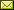## A Categorical Construction of the Real Unit Interval

 John van de Wetering

 The real unit interval is the fundamental building block for many branches of mathematics like probability theory, measure theory, convex sets and homotopy theory. However, a priori the unit interval could be considered an arbitrary choice and one can wonder if there is some more canonical way in which the unit interval can be constructed. In this paper we find such a construction by using the theory of effect algebras. We show that the real unit interval is the unique non-initial, non-final irreducible algebra of a particular monad on the category of bounded posets. The algebras of this monad carry an order, multiplication, addition and complement, and as such model much of the operations we need to do on probabilities. On a technical level, we show that both the categories of omega-complete effect algebras as well as that of omega-complete effect monoids are monadic over the category of bounded posets using Beck's monadicity theorem. The characterisation of the real unit interval then follows easily using a recent representation theorem for omega-complete effect monoids.

In Kohei Kishida: Proceedings of the Fourth International Conference on Applied Category Theory (ACT 2021), Cambridge, United Kingdom, 12-16th July 2021, Electronic Proceedings in Theoretical Computer Science 372, pp. 43–58.
Published: 3rd November 2022.

 ArXived at: http://dx.doi.org/10.4204/EPTCS.372.4 bibtex PDF
References in reconstructed bibtex, XML and HTML format (approximated).
 Comments and questions to:eptcs@eptcs.org For website issues:webmaster@eptcs.org